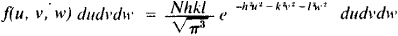# Stellar Kinematics

Also found in: Wikipedia.
The following article is from The Great Soviet Encyclopedia (1979). It might be outdated or ideologically biased.

## Stellar Kinematics

a branch of stellar astronomy that studies the regularities in the motion of various objects in the Milky Way Galaxy using statistical methods. Stellar kinematics studies the motions of stars freed from effects connected with the earth’s rotation, the earth’s revolution around the sun, nutation, precession, and so forth. The fundamental kinematic characteristics of galactic objects are their proper motions μα#x201D;, μα” and radial velocities vr, which are related to the star’s space velocity v relative to the sun by the formula

ν2 = (4.74μα”)2 + (4.74μδ”r)2 + νr2

where r is the distance from the star to the sun (here νr and ν are expressed in km/sec and r in parsecs). It is possible to characterize the motion of any group of stars in space by its average motion (motion of the group’s centroid) relative to the sun and by parameters of the distribution of residual velocities— that is, the differences of the velocities of the stars of the centroid.

Before the 20th century it was assumed that the distribution of residual velocities was random. But the first statistical studies revealed the inequality of different directions of motion for stars in our galaxy. The German astronomer K. Schwarzschild worked out a mathematical theory for the distribution of peculiar velocities, having assumed that the distribution functions for the peculiar velocities had the formThe quantities h, k, I characterize the dispersion of the component velocities in the directions of the principal axes w, v, w; N is the number of stars being investigated. The surfaces of equal density of the ends of the velocity vectors are, in the general case, triaxial ellipsoids in which the directions of the semimajor axes are close to the direction toward the center of the Milky Way Galaxy.

The ratios of the semiaxes, which are proportional to the dispersions of the residual velocities, are approximately constant for different groups of stars and amount to 1:0.6:0.5. However, their absolute values depend on which component of the Milky Way Galaxy the objects being studied belong to. Thus for stars of spectral classes O and B—typical representatives of the flat component—the root mean square velocity is approximately equal to 10 km/sec, while for objects of the spherical component it is on the order of 100 km/sec. This difference is a consequence of the dissimilar conditions of formation and ages of stars from different components.

The sun’s velocity ν0 can be determined by analyzing the motions of various groups of stars. Relative to the stars visible to the naked eye, the sun moves with a velocity of ν0 = 19.5 km/sec in the direction: right ascension 18 hr, declination approximately +30° (the so-called standard apex). Relative to certain other groups of stars, ν0 reaches 140 km/sec. The difference in the velocities of the sun relative to two centroids characterizes the mutual motion of the centroids in accordance with definite regularities. The projections upon the galactic plane of the ends of the sun’s velocity vectors for various groups of stars are situated approximately in one straight line extending in the direction of galactic longitudes 90°-270°. The explanation of this regularity was given by the Swedish astronomer B. Lindblad, who assumed that our galaxy consists of interpenetrating subsystems rotating with different velocities around the same axis passing through the center of our galaxy and perpendicular to its plane. The stars relative to which the sun has a velocity of 19.5 km/sec rotate the most rapidly. The study of the rotation of our galaxy indicates that at a distance of the sun it proceeds according to laws intermediate between the laws of the rotation of a rigid body and Kepler’s laws (closer to the latter). The influence of the differential effect of the Milky Way Galaxy’s rotation upon the components of proper motion Δμ1 and Δμ0, in the galactic coordinates/ and b and upon the radial velocities Δνr for stars within about 1 kiloparsec from the sun is expressed by formulas proposed by the Dutch astronomer J. Oort (1927):

Δνr = Ar sin 2l cos2b

Δμ1 = A cos 2l + B

Δμ0 = -Ar sin 2l sin b cos b

The rotation of the Milky Way Galaxy at the sun’s distance may be described by the following values of the parameters (Oort’s constants): A = 15 (km/sec)/kiloparsec; B = -10 (km/sec)/kiloparsec.

E. D. PAVLOVSKAIA